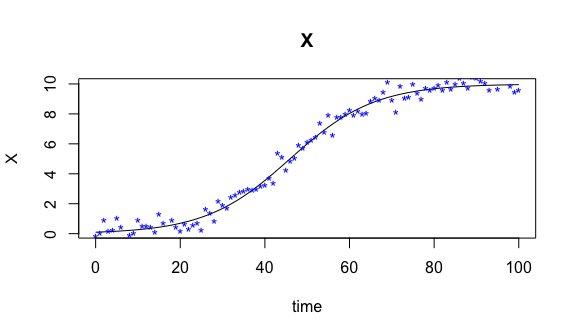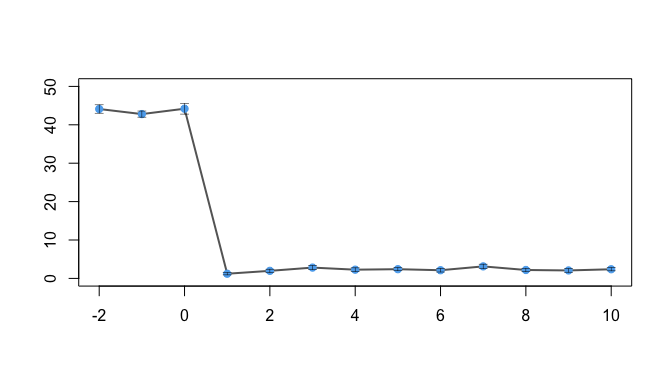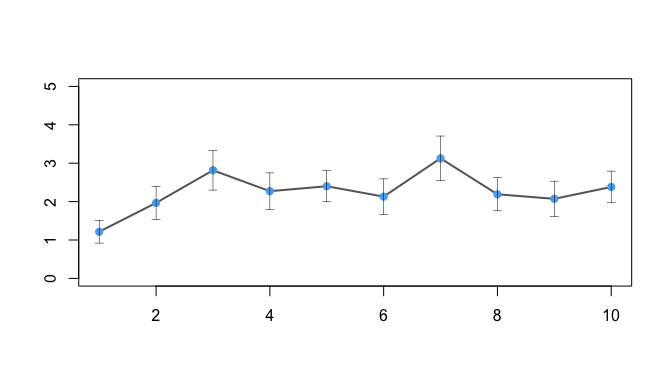# Parameter cascade method for ODE models with pCODE

knitr::opts_chunk$set( collapse = TRUE, comment = "#>" ) # Introduction Evolution of a dynamic system in time can be represented by ordinary differential equations (ODE) models consisting a set of state variables and their derivatives. For example, the state variables can be the population of different species in an ecological system, the velocities and locations of particles in a physics system, the concentration of chemicals in a reactor, or the energy of objects in a heating process. Usually, an ODE model is defned as a set of differential equations in the following form $\dot{\mathbf{x}}(t) = \mathbf{f}(\mathbf{x},\mathbf{u},t|\mathbf{\theta}) \label{eq:generalode}$ where $$\mathbf{x}(t)$$ denotes the fully or partially observed states of the system at time $$t$$. The ODE model links the first derivative, the temporal change, of the states with $$\mathbf{x}$$ itself and other influention components $$\mathbf{u}$$ through some function $$\mathbf{f}$$ defined by the parameter vector $$\mathbf{\theta}$$. First, each of $$x_{i}(t)$$ is expressed as a linear combination of basis functions $\begin{equation} x_{i}(t) = \sum_{i}^{K_{i}} c_{ik} \phi_{ik}(t) = \mathbf{c}^{\prime}_{i}\mathbf{\phi}_{i}(t) \end{equation}$ given $$K_{i}$$, the number of basis funtions, and $$\mathbf{\phi}_{i}(t)$$, the vector of basis functions evaluated at a time point t. Normally, the optimal $$K_{i}$$ is obtained through a tuning process. Larger $$K_{i}$$ leads to overfitting and wiggly estimates of ODE solutions, and insufficient basis functions cannot provide a satisfactory interpolation to capture the variation in both $$x_{i}(t)$$ and its derivative. For that matter, penalzation approach remedies the situation by imposing a smoothness constraint during the optimzation step for obtaining basis coefficients. Hence, a saturated number of basis functions can be selected without the consequence of overfitting the observations and left to be a subjective matter. As a result, $$x_{i}(t)$$ is determined by its basis coefficients $$\mathbf{c}_{i}$$, and initial values are immediately available upon the estiamtion result of $$\mathbf{c}_{i}$$. Let $$y_{ij}$$ be the j-th observation of i-th state variable of the system, where $$j = 1, ..., n_{i}$$ and $$i = 1,..., D$$. The smoothing method for obtaining $$\hat{\mathbf{c}_{i}}$$ with $$\hat{x}_{i}(t)$$ relies on the random errors defined as $\begin{equation*} e_{ij} = y_{ij} - x_{ij} \end{equation*}$ Assume $$\mathbf{e}_{i} = (e_{i1},...,e_{in_{i}})$$ has a distribution function denoted as $\begin{equation*} g(\mathbf{e}_{i}|\mathbf{\sigma}_{i}) \end{equation*}$ where $$\mathbf{\sigma}_{i}$$ represents the distribution parameters. As a start for establishing the optimization procedure, a simple objective funtion $$J(\mathbf{c}_{i}|\mathbf{\sigma}_{i})$$ is considered per the smoothing spline routine. That is, the negative log-likelihood funtion is chosen for non-normal errors, whereas the least square criterion is naturally suitable for i.i.d. normal error $$e_{ij} \sim \text{N}(0,\sigma_{i})$$. The summation of individual fitting criterion $$J(\mathbf{c}_{i}|\mathbf{\sigma}_{i})$$ over all defines a composite objective function for all dimensions. As forementioned, a saturated number of basis functions will be used which requires a penalty term in the objective function to prevent overfitting problems. suggests to use a linear differential operator for defining a roughness penalty and introuduce it to the fitting criterion. Later, utilizes the roughness penalty in estimating parameters and solutions of ODE models. Following the same technique, the penalty term is defined based on the discrepancy between the derivative and the ODE model, i.e., $\begin{equation} \int (\dot{x}_{i}(t) - f(\mathbf{x},t|\mathbf{\theta}))^{2}dt \end{equation}$ for each variable $$x_{i}$$. Multiplying the penalty with a smoothing parameter $$\lambda_{i}$$ and combining the summation of individual penalty, the complete objective function is defined to be $\begin{equation} J(\mathbf{c}|\mathbf{\theta},\mathbf{\sigma}_{i},\mathbf{\lambda}) = \sum_{i=1}^{D} \big[ g(\mathbf{e}_{i}|\mathbf{\sigma}_{i}) + \lambda_{i}\int (\dot{x}_{i}(t) - f(\mathbf{x},t|\mathbf{\theta}))^{2}dt\big] \label{eq:innerobj} \end{equation}$ where conditioning on $$\mathbf{\theta}$$ and $$\mathbf{\lambda}$$ is introduced. Additionally, the basis coefficients $$\mathbf{c}$$ become an implicit functions of the ODE parameters $$\mathbf{\theta}$$ as $$\mathbf{c}(\mathbf{\theta},\mathbf{\sigma};\mathbf{\lambda})$$. $$\mathbf{\lambda}$$ are treated as tuning parameters rather then model parameters as illustated in the difference of conditioning. Objective function~ needs to be updated in order to estiamte $$\mathbf{c}$$ given changes in $$\mathbf{\theta}$$. To clearly distinguish two sets of parameters $$\mathbf{c}$$ and $$\mathbf{\theta}$$, $$\mathbf{c}$$ will be referred to as the nuisance parameters since they are not essential for estimating the ODE model rather to interpolates the observations. On the otherhand, $$\mathbf{\theta}$$ is responsible for defining the structure of the ODE model and will be denoted as structural parameters. Often, $$\mathbf{\theta}$$ will be the primary concern given any ODE models. A lesser interest resides in the parameters $$\mathbf{\sigma}$$ of error distributions given that they influence the fitting criterion for parameter estimation. Another objective function $$H(\mathbf{\theta},\mathbf{\sigma}|\mathbf{\lambda})$$, referred to as the outter objective function, is optimized with respect only to the structural parameters $$\mathbf{\theta}$$. It is usually defined to be the negative log-likelihood function or the sum of squared errors given the distribution of random errors of the data, that is, $\begin{equation} H(\mathbf{\theta},\mathbf{\sigma}|\mathbf{\lambda}) = -\sum_{i}\text{ln }g(\mathbf{e}_{i}|\mathbf{\sigma}_{i},\mathbf{\theta},\mathbf{\lambda}) \label{eq:outterobj} \end{equation}$ where $\begin{equation*} \mathbf{e}_{i} = \mathbf{y}_{i} - \hat{\mathbf{c}}^{\prime}_{i}(\mathbf{\theta},\mathbf{\sigma};\mathbf{\lambda})\mathbf{\phi}(\mathbf{t}_{i}) \end{equation*}$ and $$\hat{\mathbf{c}}^{\prime}_{i}(\mathbf{\theta},\mathbf{\sigma};\mathbf{\lambda})$$ is the optimizer of $$J(\mathbf{c}|\mathbf{\theta},\mathbf{\sigma}_{i},\mathbf{\lambda})$$ given current $$\mathbf{\theta}$$ and $$\lambda_{i}$$. $$H(\mathbf{\theta},\mathbf{\sigma}|\mathbf{\lambda})$$ does not need to have a regularization term given that basis coefficients $$\mathbf{c}_{i}$$ have already been regularized. The profiling estimation procedure entails two nested optimizations that are controled by the smoothing parameters $$\mathbf{\lambda}$$, where the inner optimization depends on the $$\mathbf{\lambda}$$ through the outter one. As a result, fitting the data is not marginalized from estimating structural prameters. The stream of dependencies defined a parameter cascade which offers great accuracy and efficiency in estimating ODE models. To take full advatanges of the parameter cascade method, pCODE package provides functions that are able to apply the forementioned methodology for estimating ODE models. # Package functions ## Parameter estimation: pcode The main function to perform parameter cascade method is pcode in the package. This is a generic wrapper for handling different scenarios in terms of objective functions, likelihood or sum of squared errors. First, we can focus on the situation where $$e_{ij}\sim\text{N}(0,\sigma^{2}_{i})$$. In fact, both inner and outter objective function are calculated based on a vector of residuals. The first part of inner objective is easily recognized as the residual from fitting the observation with basis funtions under the non-linear least square (NLS) problem. If the second part, the integral, is approximated by the composite Simpson’s rule, then the approximation can be written in a quadratic form and as the inner product between a vector of residual-like quantities to itself. Stringing the mentioned two vectors together, and the concatenation of them from all dimensions will produce a vector of residuals. Hence, the optimization of inner objective function adheres the NLS framework for a given $$\mathbf{\theta}$$. As a result, the popular Levenberg-Marquart algorithm is used to obtain an esimate $$\hat{\mathbf{c}}^{\prime}_{i}(\mathbf{\theta},\mathbf{\sigma};\mathbf{\lambda})$$, and pcode employes the funtion lsqnonlin from PRACMA. The optimization of outter objective function appears to be exactly a NLS problem. However, the profiled estiamtion strategy appends an extra layer of optimization to each update of outter estimation which characterizes a nested optimization problem. It is applicable to apply Levenberg-Marquart algorithm again to the outter objective, however the computational effort is much greater than that of inner optimization. ## Tuning $$\lambda$$: tunelambda The penalty parameter $$\mathbf{\lambda}$$ can be manually adjusted for the optimization procedure with function , or it can be determined from a grid search based on a k-fold cross validatoin(CV) criterion. We define the CV score of any set of $$\mathbf{\lambda}$$ to be $\text{CV}(\mathbf{\lambda}) = \sum_{i=1}^{D}\sum_{j=1}^{v} ( y_{i}(t^{\ast}_{j}) - \hat{x}_{i}(t^{\ast}_{j}))^{2}$ where $$v$$ indicates to the total number of points kept from estimation and $$t^{\ast}_{j}$$, for $$j = 1,..., v$$, corresponds to those points. The $$t^{\ast}_{j}$$’s are set to be the same across each dimension $$d$$ of the ODE model. The points kept away from estimation are defined by the number of folds and the portion of points in each fold. That is, a certain portion of points are kept within each fold of the entire vector of observation points. ## Variance estimation of $$\mathbf{\theta}$$: bootsvar and deltavar All the functions of this packages are derivative-free, hence the variance of structural parameters is numerically approximated. The first option is to use the bootstrap variance estimator. Given the estimation of both parameters $$\mathbf{\theta}$$ and $$\mathbf{c}$$, we are able to solve the ODE directly with estimated initial value $$\hat{\mathbf{x}}(t_{0})$$. Hence, bootstrap samples of the ODE model can be simulated based on the estimated distribution of errors $$\mathbf{e}_{i}$$ for each dimension. As an alternative to the boostrap variance estimator, the package also offers another numeric estimator for the variance of structural parameters. has developed the approximation to $$Var(\mathbf{\hat{\theta}}(\mathbf{y}))$$ via the delta-method. The resulting approximation is of the form $\begin{equation*} Var(\hat{\mathbf{\theta}}(\mathbf{y})) \approx \bigg[ \frac{d\mathbf{\hat{\theta}}}{d\mathbf{y}} \bigg] \mathbf{\Sigma} \bigg[ \frac{d\mathbf{\hat{\theta}}}{d\mathbf{y}} \bigg]^{\prime} \end{equation*}$ where $$\frac{d\mathbf{\hat{\theta}}}{d\mathbf{y}}$$ is obtained as $\begin{equation} \label{eq:dtheta_dy} \frac{d\mathbf{\hat{\theta}}}{d\mathbf{y}} = - \bigg[\frac{\partial^{2}H}{\partial\mathbf{\theta}^2}\bigg\vert_{\mathbf{\hat{\theta}}(\mathbf{y})} \bigg]^{-1} \bigg[ \frac{\partial^{2}H}{\partial\mathbf{\theta}\partial\mathbf{y}}\bigg\vert_{\mathbf{\hat{\theta}}(\mathbf{y})} \bigg] \end{equation}$ and $$\mathbf{\Sigma}$$ is a $$N*N$$ matrix where the diagonal elements correspond to the variances for each observation. $$N$$ is the total number of observation summing over all dimensions of $$\mathbf{y}$$ . Then the estimation of variance relies on the computation of (). The partial derivatives are approximated by the finite difference method, i.e., $\begin{equation*} \bigg[\frac{\partial^{2}H}{\partial\mathbf{\theta}_{u}^2}\bigg\vert_{\mathbf{\hat{\theta}}(\mathbf{y})} \bigg] \approx \frac{H(\hat{\mathbf{\theta}}_{u+}(\mathbf{y})|\mathbf{\lambda}) - 2H(\hat{\mathbf{\theta}}(\mathbf{y})|\mathbf{\lambda}) + H(\hat{\mathbf{\theta}}_{u-}(\mathbf{y})|\mathbf{\lambda})}{\Delta^{2}} \end{equation*}$ where $$\hat{\mathbf{\theta}}_{u+}(\mathbf{y})$$ and $$\hat{\mathbf{\theta}}_{u-}(\mathbf{y})$$ indicate the addition and subtraction of stepsize $$\Delta$$ to the u-th structural parameter estimate $$\hat{\mathbf{\theta}}(\mathbf{y})$$. The mixed partial derivatives are approximated as the following $\begin{equation*} \bigg[\frac{\partial^{2}H}{\partial\mathbf{\theta}_{u}\partial\mathbf{\theta}_{v}}\bigg\vert_{\mathbf{\hat{\theta}}(\mathbf{y})} \bigg] \approx \frac{H(\hat{\mathbf{\theta}}_{u+,v+}(\mathbf{y})) - H(\hat{\mathbf{\theta}}_{u-,v+}(\mathbf{y})) - H(\hat{\mathbf{\theta}}_{u+,v-}(\mathbf{y})) +H(\hat{\mathbf{\theta}}_{u-,v-}(\mathbf{y}))}{4\Delta^{2}} \end{equation*}$ Given any fixed arugument $$\mathbf{\theta}$$ for the outter objective function $$H(\mathbf{\theta},\mathbf{\sigma}|\mathbf{\lambda})$$, its evaluation involves the profiled estimation of $$\mathbf{c}(\mathbf{\theta},\mathbf{\sigma};|\mathbf{\lambda})$$ and returns solely the likelihood or the sum of squared errors. Hence, individual evaluations of $$H(\mathbf{\theta},\mathbf{\sigma}|\mathbf{\lambda})$$ used in numeric approximation is obtained in the following steps: Approximation to the second term $$\frac{\partial^{2}H}{\partial\mathbf{\theta}\partial\mathbf{y}}\bigg\vert_{\mathbf{\hat{\theta}}(\mathbf{y})}$$ utilizes the finite difference method as well. After evaluating $$H(\mathbf{\theta},\mathbf{\sigma}|\mathbf{\lambda})$$ given $$\mathbf{\theta}$$, the mixed partial derivative is calculated by moving the particular observation up or down by some stepsize $$\Delta_{y}$$. That is, $\begin{equation*} \bigg[\frac{\partial^{2}H}{\partial\mathbf{\theta}_{u}\partial y_{v}}\bigg\vert_{\mathbf{\hat{\theta}}(\mathbf{y})} \bigg] \approx \frac{H(\hat{\mathbf{\theta}}_{u+},\mathbf{y}_{v+}) - H(\hat{\mathbf{\theta}}_{u+},\mathbf{y}_{v-}) - H(\hat{\mathbf{\theta}}_{u-},\mathbf{y}_{v+}) +H(\hat{\mathbf{\theta}}_{u-},\mathbf{y}_{v-})}{4\Delta\Delta_{y}} \end{equation*}$ where $$\mathbf{y}_{v+}$$ and $$\mathbf{y}_{v-}$$ represent moving up and down v-th observation by stepsize $$\Delta_{y}$$ in the last step of evaluating $$H(\mathbf{\theta},\mathbf{\sigma}|\mathbf{\lambda})$$. # A simple example A simple illustration uses an one-dimensional ODE model $\dot{X} = \theta X (1-\frac{X}{10})$ The following code defines the forementioned model that will be provided for pcode for estimating parameters and ode for obtaining numeric solution: #load dependencies library(pCODE) library(deSolve) library(fda) library(MASS) library(pracma) library(Hmisc) #set seed for reproducibility set.seed(123) ode.model <- function(t,state,parameters){ with(as.list(c(state,parameters)),{ dX <- theta*X*(1-X/10) return(list(dX))})} Let $$\theta = 0.1$$ and $$X(0) = 0.1$$ #define model parameters model.par <- c(theta = c(0.1)) #define state initial value state <- c(X = 0.1) Given an observation period of $$[0,100]$$, random noise errors are added to the ODE solution with a Normal distribution $$\text{N}(0,0.5^{2})$$. Observations are generated as follows: times <- seq(0,100,length.out=101) mod <- ode(y=state,times=times,func=ode.model,parms = model.par) nobs <- length(times) scale <- 0.5 noise <- scale * rnorm(n = nobs, mean = 0 , sd = 1) observ <- mod[,2] + noise Subsequently, we can visualize the observations along the true solution of this simple ODE model as #plot simulated data against generating model plot(mod,ylab=names(state)) #curve points(times, observ,pch='*',col='blue') #observationFirst, a basis object needs to be defined by create.bspline.basis from fda package for smoothing observations. A B-spline basis is created given 21 konts including both interior and boundary knots acorss observation interval $$(0,100)$$. The order of basis functions is 4, so there is a total of 23 basis functions. #Generate basis object for interpolation and as argument of pcode #21 konts equally spaced within [0,100] knots <- seq(0,100,length.out=21) #order of basis functions norder <- 4 #number of basis funtions nbasis <- length(knots) + norder - 2 #creating Bspline basis basis <- create.bspline.basis(c(0,100),nbasis,norder,breaks = knots) To perform parameter cascade method for estimating both structural and nuisance parameters, one can use pcode in the following way #parameter estimation pcode.result <- pcode(data = observ, time = times, ode.model = ode.model, par.initial = 0.3, par.names = 'theta',state.names = 'X', basis.list = basis, lambda = 1e2) The structural parameter and nuisance parameter estiamtes can be called by pcode.result$structural.par
#>      theta
#> 0.09995229
pcode.result$nuisance.par #>  0.1232160 0.1550332 0.1903906 0.2729993 0.4284428 0.6134020 #>  1.0222183 1.6891098 2.5351231 3.5543293 4.8116926 6.0699739 #>  7.2145361 8.0734588 8.7456720 9.2110485 9.4866269 9.7101657 #>  9.8736650 9.9650449 10.0098433 9.9950749 9.9846698 The true variance for data generating parameter $$\theta$$ is $$1.003\mathrm{e}{-5}$$, and we can then compare the performance deltavar for estimating $$\text{var}(\theta)$$. deltavar(data = observ, time = times, ode.model = ode.model, par.initial = 0.3, par.names = 'theta',state.names = 'X', basis.list = basis, lambda = 1e2, stepsize = 1e-5,y_stepsize = 1e-5) #>  "#######################################" #>  "Starting optimization" #>  "#######################################" #>  "Current iteration: 1" #>  "Current function value: 124.675163" #>  "Current iteration: 2" #>  "Current function value: 33.572556" #>  "Current iteration: 3" #>  "Current function value: 31.913573" #>  "Current iteration: 4" #>  "Current function value: 20.006666" #>  "Current iteration: 5" #>  "Current function value: 20.003467" #>  "Current iteration: 6" #>  "Current function value: 20.003467" #>  "Current iteration: 7" #>  "Current function value: 20.003467" #>  9.923318e-06 The variance estimator give sexcellent estimates of the true variance of structural parameter. tunelambda returns a matrix of cross-validation scores for each replication given a certain $$\lambda$$. Based on the replicates, score averages and standard deviations can be calculated for comparison betweens candidates of $$\lambda$$: #Tune lambda based on k-fold cross-validation cv.result <- tunelambda(data = observ, time = times, ode.model = ode.model, par.initial = 0.3, par.names = 'theta',state.names = 'X', basis.list = basis, lambda_grid = 10^(-3:10),cv_portion = .01, rep = 20, kfolds = 5) cv.means <- apply(cv.result$cv.score, 1, mean)
cv.sd    <- apply(cv.result\$cv.score, 1, sd)/sqrt(20)
plot(-2:10, cv.means[2:14], type = "l", lwd = 2, col = gray(0.4),ylim= c(-0,50),ylab='',xlab='')
errbar(-2:10, cv.means[2:14], cv.means[2:14] + cv.sd[2:14], cv.means[2:14] - cv.sd[2:14], add = TRUE, col = "steelblue2", pch = 19,
lwd = 0.5)

plot(1:10, cv.means[5:14], type = "l", lwd = 2, col = gray(0.4),ylim= c(-0,5),ylab='',xlab='')
errbar(1:10, cv.means[5:14], cv.means[5:14] + cv.sd[5:14], cv.means[5:14] - cv.sd[5:14], add = TRUE, col = "steelblue2", pch = 19,
lwd = 0.5)The search grid for $$\lambda$$ is $$10^{-2:10}$$ in this case. The CV scores, as illustrated in , indicate that any $$\lambda$$ less than $$1$$ are not optimal for the model Instead, we can focus on a subset of candidates for which the CV scores are considered to be reasonably acceptable. Based on the cross-validation plot, $$\lambda = 10$$ seems to be the most optimal.